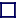### ALPHA DECAY

The emission of an alpha particle (a helium nucleus consisting of two protons bound to two neutrons) by a nuclide is impossible unless the mass of the daughter product plus the mass of the emitted alpha particle is less than that of the parent nuclide. The greater this mass difference, the shorter the halflife. If alpha decay did not occur, all isobars would have at least one stable member. Alpha decay is energetically favoured for nuclides with Atomic Weight (A) greater than about 150. After alpha decay, the Atomic Number (Z) decreases by 2 and the Atomic Weight by 4 (e.g. Astatine-214 becomes bismuth-210). It will be observed that nuclides of Atomic Weight between A=215 and A=222 all decay by alpha decay and all have relatively short half-lives (between 30ms and 100ps) compared to those with higher Atomic Weight (which have halflives up to 1000 Million years). Unlike electrons in beta decay the alpha particles are emitted with very specific energies. For nuclides with atomic weights between 144 and 206, the lower the energy of the emitted alpha particle, the longer will be the half life.An alpha particle: 2 protons + 2 neutrons

Alpha particle emission, like fission and fusion, involves barrier penetration, or the tunnelling of the alpha particle through a potential barrier. Because the width of this barrier can assume various proportions for different nuclides, the half-life for alpha decay can vary over 24 orders of magnitude from 2×1010 years for thorium-232 (which decays into radium-228) to 4.3×10-7 seconds for polonium-212 (which becomes Lead-208).

The disintegration energy (sometimes called the Q-value) liberated by alpha decay is between 2MeV and 9MeV. When the energy difference is less than 2MeV, the decay is either not observed or the halflife is incredibly long because the-particle has to traverse a very wide energy barrier. This occurs for some nuclides with A<150 and with bismuth-209 (halflife 2×1017 years).

The binding energy lost by the process of emitting an alpha particle is shared (in-equally) by the alpha particle and its' daughter nucleus. The alpha particle and daughter nucleus must separate with equal but opposite momenta, but because the daughter nucleus has a much greater mass than the alpha particle, the alpha particle acquires most of the energy, leaving the daughter nucleus with a slight recoil velocity (and hence energy). The energies acquired by both alpha particle and daughter nuclide are well defined.

Shown is astatine-213 decaying by alpha decay into the stable bismuth-209.